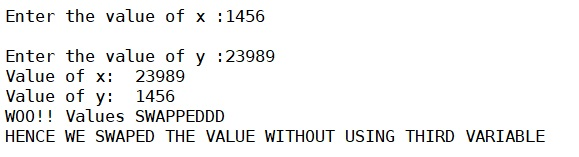# Python program for swapping the value of two integers without third variable

Here, we are going to learn how to swap the value of two integers without using third variable in Python?
Submitted by Anuj Singh, on June 24, 2019

In this article, we will learn how to swap the value of two integers without using a different (third) variable? In this problem, we are about to explore a new feature of the tuple in python.

One simple step, two different variables and it's all done.

So here is the code:

```x = int(input("Enter the value of x :"))
y = int(input("Enter the value of y :"))

(x,y) = (y,x)

print('Value of x: ', x, '\nValue of y: ', y, '\nWOO!! Values SWAPPEDDD')

print('HENCE WE SWAPED THE VALUE WITHOUT USING THIRD VARIABLE')
```

Output:Preparation